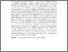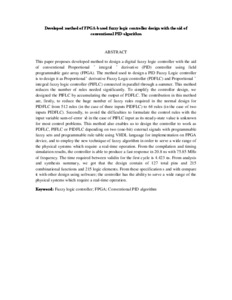# Developed method of FPGA-based fuzzy logic controller design with the aid of conventional PID algorithm

## Citation

Obaid, Zeyad Assi and Sulaiman, Nasri and Hamidon, Mohd Nizar (2009) Developed method of FPGA-based fuzzy logic controller design with the aid of conventional PID algorithm. Australian Journal of Basic and Applied Sciences, 3 (3). pp. 2724-2740. ISSN 1991-8178

## Abstract

This paper proposes developed method to design a digital fuzzy logic controller with the aid of conventional Proportional – integral – derivative (PID) controller using field programmable gate array (FPGA). The method used to design a PID Fuzzy Logic controller is to design it as Proportional– derivative Fuzzy Logic controller (PDFLC) and Proportional –integral fuzzy logic controller (PIFLC) connected in parallel through a summer. This method reduces the number of rules needed significantly. To simplify the controller design, we designed the PIFLC by accumulating the output of PDFLC. The contribution in this method are, firstly, to reduce the huge number of fuzzy rules required in the normal design for PIDFLC from 512 rules (in the case of three inputs PIDFLC) to 64 rules (in the case of two inputs PIDFLC). Secondly, to avoid the difficulties to formulate the control rules with the input variable sum-of-error Σe in the case of PIFLC input as its steady-state value is unknown for most control problems. This method also enables us to design the controller to work as PDFLC, PIFLC or PIDFLC depending on two (one-bit) external signals with programmable fuzzy sets and programmable rule table using VHDL language for implementation on FPGA device, and to employ the new technique of fuzzy algorithm in order to serve a wide range of the physical systems which require a real-time operation. From the compilation and timing simulation results, the controller is able to produce a fast response in 20.8 ns with 75.85 MHz of frequency. The time required between validin for the first cycle is 4.423 ns. From analysis and synthesis summary, we got that the design contain of 127 total pins and 215 combinational functions and 215 logic elements. From these specification s and with compare it with other design using software; the controller has the ability to serve a wide range of the physical systems which require a real-time operation.Preview
Text (Abstract)
Developed method of FPGA-based fuzzy logic controller design with the aid of conventional PID algorithm.pdfView Item